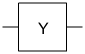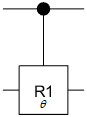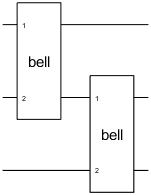## Types of Quantum Gates

Note

Installation Required: This functionality requires MATLAB Support Package for Quantum Computing.

This topic provides a list functions that you can use to create quantum gates in MATLAB®. Quantum gates are reversible and have unitary matrix representations.

### Creation Functions for `SimpleGate` Objects

#### Gates on One Target Qubit

Creation FunctionGate NameNo. of QubitsMatrix RepresentationProperties`hGate` Hadamard gate1$\frac{1}{\sqrt{2}}\left[\begin{array}{cc}1& 1\\ 1& -1\end{array}\right]$
• Traceless

• Involutory`idGate`Identity gate1$\left[\begin{array}{cc}1& 0\\ 0& 1\end{array}\right]$
• Identity

• Involutory`xGate`Pauli X gate1$\left[\begin{array}{cc}0& 1\\ 1& 0\end{array}\right]$
• Traceless

• Involutory

• Pauli group`yGate`Pauli Y gate1$\left[\begin{array}{cc}0& -i\\ i& 0\end{array}\right]$
• Traceless

• Involutory

• Pauli group`zGate`Pauli Z gate1$\left[\begin{array}{cc}1& 0\\ 0& -1\end{array}\right]$
• Traceless

• Involutory

• Pauli group

#### Rotation Gates

Creation FunctionGate NameNo. of QubitsMatrix RepresentationProperties`rxGate`x-axis rotation gate1$\left[\begin{array}{cc}\mathrm{cos}\left(\frac{\theta }{2}\right)& -i\mathrm{sin}\left(\frac{\theta }{2}\right)\\ -i\mathrm{sin}\left(\frac{\theta }{2}\right)& \mathrm{cos}\left(\frac{\theta }{2}\right)\end{array}\right]$
• Special unitary (determinant is 1)

• Continuous parameter $\theta$ with period $\text{4}\pi$`ryGate`y-axis rotation gate1$\left[\begin{array}{cc}\mathrm{cos}\left(\frac{\theta }{2}\right)& -\mathrm{sin}\left(\frac{\theta }{2}\right)\\ \mathrm{sin}\left(\frac{\theta }{2}\right)& \mathrm{cos}\left(\frac{\theta }{2}\right)\end{array}\right]$
• Special unitary (determinant is 1)

• Continuous parameter $\theta$ with period $\text{4}\pi$`rzGate`z-axis rotation gate1$\left[\begin{array}{cc}\mathrm{exp}\left(-i\text{\hspace{0.17em}}\frac{\theta }{2}\right)& 0\\ 0& \mathrm{exp}\left(i\text{\hspace{0.17em}}\frac{\theta }{2}\right)\end{array}\right]$
• Special unitary (determinant is 1)

• Continuous parameter $\theta$ with period $\text{4}\pi$`r1Gate`z-axis rotation gate with global phase1$\left[\begin{array}{cc}1& 0\\ 0& \mathrm{exp}\left(i\text{\hspace{0.17em}}\theta \right)\end{array}\right]$
• Continuous parameter $\theta$ with period $2\pi$`sGate`S gate1$\left[\begin{array}{cc}1& 0\\ 0& i\end{array}\right]$`siGate`Inverse S gate1$\left[\begin{array}{cc}1& 0\\ 0& -i\end{array}\right]$`tGate`T gate1$\left[\begin{array}{cc}1& 0\\ 0& \frac{1+i}{\sqrt{2}}\end{array}\right]$`tiGate`Inverse T gate1$\left[\begin{array}{cc}1& 0\\ 0& \frac{1-i}{\sqrt{2}}\end{array}\right]$

#### Gates with One Control Qubit and One Target Qubit

Creation FunctionGate NameNo. of QubitsMatrix RepresentationProperties`chGate` Controlled Hadamard gate2$\left[\begin{array}{cccc}1& 0& 0& 0\\ 0& 1& 0& 0\\ 0& 0& \frac{1}{\sqrt{2}}& \frac{1}{\sqrt{2}}\\ 0& 0& \frac{1}{\sqrt{2}}& -\text{\hspace{0.17em}}\frac{1}{\sqrt{2}}\end{array}\right]$
• Involutory`cxGate` or `cnotGate`

Controlled X or CNOT gate2$\left[\begin{array}{cccc}1& 0& 0& 0\\ 0& 1& 0& 0\\ 0& 0& 0& 1\\ 0& 0& 1& 0\end{array}\right]$
• Involutory`cyGate`Controlled Y gate2$\left[\begin{array}{cccc}1& 0& 0& 0\\ 0& 1& 0& 0\\ 0& 0& 0& -i\\ 0& 0& i& 0\end{array}\right]$
• Involutory`czGate`Controlled Z gate2$\left[\begin{array}{cccc}1& 0& 0& 0\\ 0& 1& 0& 0\\ 0& 0& 1& 0\\ 0& 0& 0& -1\end{array}\right]$
• Involutory

• Swapping control and target qubits does not change gate operation

#### Gate That Swap States of Two Qubits

Creation FunctionGate NameNo. of QubitsMatrix RepresentationProperties`swapGate`Swap gate2$\left[\begin{array}{cccc}1& 0& 0& 0\\ 0& 0& 1& 0\\ 0& 1& 0& 0\\ 0& 0& 0& 1\end{array}\right]$
• Involutory

• Swapping the two target qubits does not change gate operation

#### Controlled Rotation Gates

Creation FunctionGate NameNo. of QubitsMatrix RepresentationProperties`crxGate`Controlled x-axis rotation gate2$\left[\begin{array}{cccc}1& 0& 0& 0\\ 0& 1& 0& 0\\ 0& 0& \mathrm{cos}\left(\frac{\theta }{2}\right)& -i\mathrm{sin}\left(\frac{\theta }{2}\right)\\ 0& 0& -i\mathrm{sin}\left(\frac{\theta }{2}\right)& \mathrm{cos}\left(\frac{\theta }{2}\right)\end{array}\right]$
• Continuous parameter $\theta$ with period $\text{4}\pi$`cryGate`Controlled y-axis rotation gate2$\left[\begin{array}{cccc}1& 0& 0& 0\\ 0& 1& 0& 0\\ 0& 0& \mathrm{cos}\left(\frac{\theta }{2}\right)& -\mathrm{sin}\left(\frac{\theta }{2}\right)\\ 0& 0& \mathrm{sin}\left(\frac{\theta }{2}\right)& \mathrm{cos}\left(\frac{\theta }{2}\right)\end{array}\right]$
• Continuous parameter $\theta$ with period $\text{4}\pi$`crzGate`Controlled z-axis rotation gate2$\left[\begin{array}{cccc}1& 0& 0& 0\\ 0& 1& 0& 0\\ 0& 0& \mathrm{exp}\left(-i\text{\hspace{0.17em}}\frac{\theta }{2}\right)& 0\\ 0& 0& 0& \mathrm{exp}\left(i\text{\hspace{0.17em}}\frac{\theta }{2}\right)\end{array}\right]$
• Continuous parameter $\theta$ with period $\text{4}\pi$`cr1Gate`Controlled z-axis rotation gate with global phase2$\left[\begin{array}{cccc}1& 0& 0& 0\\ 0& 1& 0& 0\\ 0& 0& 1& 0\\ 0& 0& 0& \mathrm{exp}\left(i\text{\hspace{0.17em}}\theta \right)\end{array}\right]$
• Continuous parameter $\theta$ with period $2\pi$

• Swapping control and target qubits does not change gate operation

#### Controlled Controlled X Gate

Creation FunctionGate NameNo. of QubitsMatrix RepresentationProperties`ccxGate`Controlled controlled X gate (CCNOT or Toffoli gate)3$\left[\begin{array}{cccccccc}1& 0& 0& 0& 0& 0& 0& 0\\ 0& 1& 0& 0& 0& 0& 0& 0\\ 0& 0& 1& 0& 0& 0& 0& 0\\ 0& 0& 0& 1& 0& 0& 0& 0\\ 0& 0& 0& 0& 1& 0& 0& 0\\ 0& 0& 0& 0& 0& 1& 0& 0\\ 0& 0& 0& 0& 0& 0& 0& 1\\ 0& 0& 0& 0& 0& 0& 1& 0\end{array}\right]$
• Involutory

• Swapping the two control qubits does not change gate operation

#### Ising Coupling Gates

Creation FunctionGate NameNo. of QubitsMatrix RepresentationProperties`rxxGate`Ising XX coupling gate2$\left[\begin{array}{cccc}\mathrm{cos}\left(\frac{\theta }{2}\right)& 0& 0& -i\mathrm{sin}\left(\frac{\theta }{2}\right)\\ 0& \mathrm{cos}\left(\frac{\theta }{2}\right)& -i\mathrm{sin}\left(\frac{\theta }{2}\right)& 0\\ 0& -i\mathrm{sin}\left(\frac{\theta }{2}\right)& \mathrm{cos}\left(\frac{\theta }{2}\right)& 0\\ -i\mathrm{sin}\left(\frac{\theta }{2}\right)& 0& 0& \mathrm{cos}\left(\frac{\theta }{2}\right)\end{array}\right]$
• Special unitary (determinant is 1)

• Continuous parameter $\theta$ with period $\text{4}\pi$

• Swapping the two target qubits does not change gate operation`ryyGate`Ising YY coupling gate2$\left[\begin{array}{cccc}\mathrm{cos}\left(\frac{\theta }{2}\right)& 0& 0& i\mathrm{sin}\left(\frac{\theta }{2}\right)\\ 0& \mathrm{cos}\left(\frac{\theta }{2}\right)& -i\mathrm{sin}\left(\frac{\theta }{2}\right)& 0\\ 0& -i\mathrm{sin}\left(\frac{\theta }{2}\right)& \mathrm{cos}\left(\frac{\theta }{2}\right)& 0\\ i\mathrm{sin}\left(\frac{\theta }{2}\right)& 0& 0& \mathrm{cos}\left(\frac{\theta }{2}\right)\end{array}\right]$
• Special unitary (determinant is 1)

• Continuous parameter $\theta$ with period $\text{4}\pi$

• Swapping the two target qubits does not change gate operation`rzzGate`Ising ZZ coupling gate2$\left[\begin{array}{cccc}\mathrm{exp}\left(-i\text{\hspace{0.17em}}\frac{\theta }{2}\right)& 0& 0& 0\\ 0& \mathrm{exp}\left(i\text{\hspace{0.17em}}\frac{\theta }{2}\right)& 0& 0\\ 0& 0& \mathrm{exp}\left(i\text{\hspace{0.17em}}\frac{\theta }{2}\right)& 0\\ 0& 0& 0& \mathrm{exp}\left(-i\text{\hspace{0.17em}}\frac{\theta }{2}\right)\end{array}\right]$
• Special unitary (determinant is 1)

• Continuous parameter $\theta$ with period $\text{4}\pi$

• Swapping the two target qubits does not change gate operation

### Creation Functions for `CompositeGate` Objects

#### Composite and Specialized Gates

Creation FunctionGate NameNo. of QubitsGate SymbolEquivalent Internal GatesMatrix Representation
`compositeGate`Composite gateVaries

Example: Quantum circuit with two composite gates named `"bell"`. The equivalent internal gates of each composite gate are a Hadamard gate and a controlled X gate.`$\frac{1}{2}\left[\begin{array}{cccccccc}1& 0& 1& 0& 1& 0& 1& 0\\ 0& 1& 0& 1& 0& 1& 0& 1\\ 0& 1& 0& -1& 0& 1& 0& -1\\ 1& 0& -1& 0& 1& 0& -1& 0\\ 1& 0& 1& 0& -1& 0& -1& 0\\ 0& 1& 0& 1& 0& -1& 0& -1\\ 0& -1& 0& 1& 0& 1& 0& -1\\ -1& 0& 1& 0& 1& 0& -1& 0\end{array}\right]$`
`qftGate`Quantum Fourier transform (QFT) gateVaries

Example: Quantum Fourier transform gate on three qubits. The equivalent internal gates are Hadamard gates, R1 gates, and a swap gate.`$\begin{array}{l}\frac{1}{\sqrt{8}}\left[\begin{array}{cccccccc}1& 1& 1& 1& 1& 1& 1& 1\\ 1& \omega & {\omega }^{2}& {\omega }^{3}& {\omega }^{4}& {\omega }^{5}& {\omega }^{6}& {\omega }^{7}\\ 1& {\omega }^{2}& {\omega }^{4}& {\omega }^{6}& 1& {\omega }^{2}& {\omega }^{4}& {\omega }^{6}\\ 1& {\omega }^{3}& {\omega }^{6}& \omega & {\omega }^{4}& {\omega }^{7}& {\omega }^{2}& {\omega }^{5}\\ 1& {\omega }^{4}& 1& {\omega }^{4}& 1& {\omega }^{4}& 1& {\omega }^{4}\\ 1& {\omega }^{5}& {\omega }^{2}& {\omega }^{7}& {\omega }^{4}& \omega & {\omega }^{6}& {\omega }^{3}\\ 1& {\omega }^{6}& {\omega }^{4}& {\omega }^{2}& 1& {\omega }^{6}& {\omega }^{4}& {\omega }^{2}\\ 1& {\omega }^{7}& {\omega }^{6}& {\omega }^{5}& {\omega }^{4}& {\omega }^{3}& {\omega }^{2}& \omega \end{array}\right]\\ \text{where}\text{\hspace{0.17em}}\omega =\mathrm{exp}\left(\frac{2\pi i}{8}\right)\end{array}$`
`mcxGate`Multi-controlled X gateVaries

Example: Multi-controlled X gate with three control qubits, one target qubit, and no ancilla qubit. The equivalent internal gates are Hadamard gates, controlled R1 gates, and controlled X gates.`$\left[\begin{array}{ccccccc}1& 0& 0& 0& 0& 0& 0\\ 0& 1& 0& 0& 0& 0& 0\\ 0& 0& \ddots & 0& 0& 0& 0\\ 0& 0& 0& 1& 0& 0& 0\\ 0& 0& 0& 0& 1& 0& 0\\ 0& 0& 0& 0& 0& 0& 1\\ 0& 0& 0& 0& 0& 1& 0\end{array}\right]$`